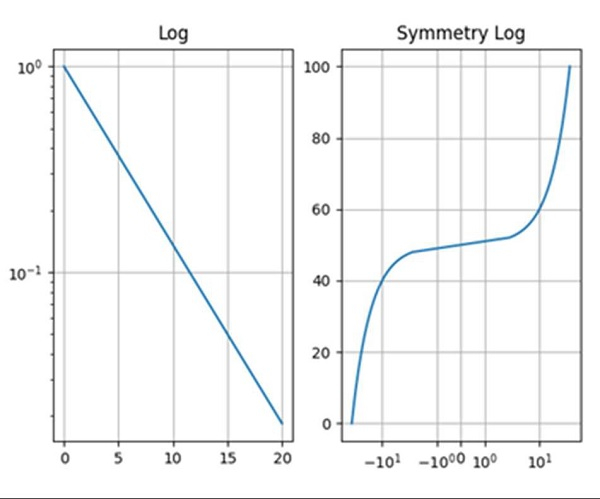# What is the difference between 'log' and 'symlog' in matplotlib?

Log helps to make a plot with log scaling on both the X and Y axis, whereas symlog (symmetric log) is used for axis scaling.

## Steps

• First, we can adjust the subplot layout parameters.

• Return an evenly spaced value (t) within a given interval, using the numpy.arrange() method.

• Add a subplot to the current figure, with nrows = 1, ncols = 2 and current index is 1.

• Make a plot with log scaling on the Y axis, using the semilogy() method.

• Set the title for the axes, using the plt.title() method.

• Configure the grid lines, using the grid(True) method.

• Create two evenly spaced values within a given interval using the numpy.arrange() method.

• Add a subplot to the current figure with nrows = 1, ncols = 2 and current index is 2.

• Plot using the plt.plot() method with two lists that have been created in step 7.

• Set the X-axis scale, using xscale.

• Set the title for the axes, using the plt.title() method.

• Configure the grid lines, using the grid(True) method.

• To show the figure, use the plt.show() method.

## Example

import numpy as np
import matplotlib.pyplot as plt

t = np.arange(0.01, 20.0, 0.01)

# log y axis
plt.subplot(121)
plt.semilogy(t, np.exp(-t/5.0))
plt.title('Log')
plt.grid(True)

x = np.arange(-50.0, 50.0, 0.01)
y = np.arange(0, 100.0, 0.01)
plt.subplot(122)
plt.plot(x, y)
plt.xscale('symlog')
plt.title('Symmetry Log')
plt.grid(True)

plt.show()

## Output